# Andhra Pradesh SSC Board Question Paper for Class 10th Maths Paper 1 2016 In PDF

## AP SSC or 10th Class Question Paper Mathematics Paper 1 English Medium 2016 with Solutions – Free Download

Andhra Pradesh SSC (Class 10) Maths 2016 question paper 1 with solutions are available here in a downloadable pdf format and also in the text, so that the students can easily access them. Along with the solutions, Maths question paper 1 2016 Class 10 SSC is also available for reference. Students are able to access all the Andhra Pradesh board previous year maths question papers here. AP 10th Class Mathematics Question Paper 2016 Paper 1 can be downloaded easily and students can practice and verify the answers provided by BYJU’S. Solving 2016 Maths question paper 1 for Class 10 will help the students to predict what type of questions will appear in the exam.

### QUESTION PAPER CODE 15E(A)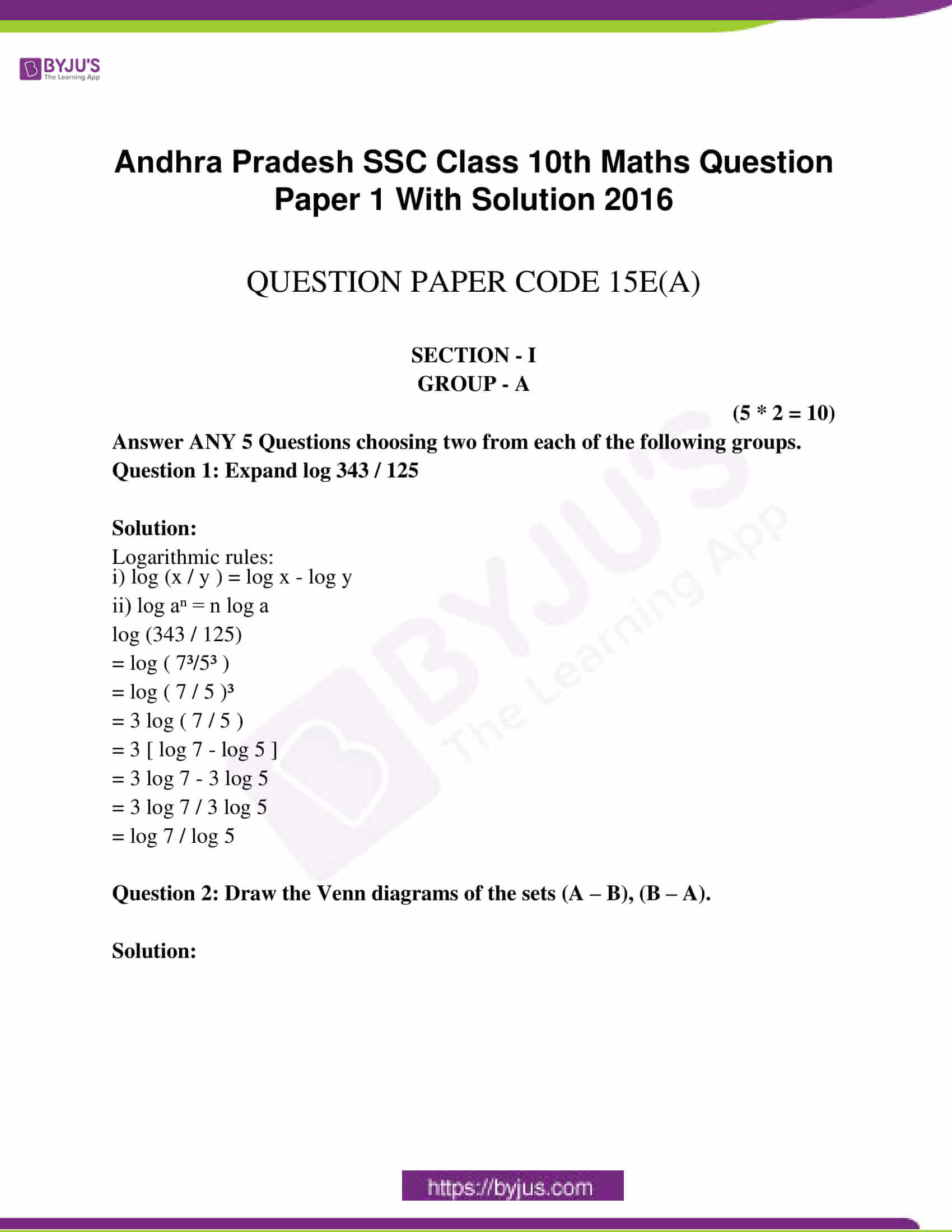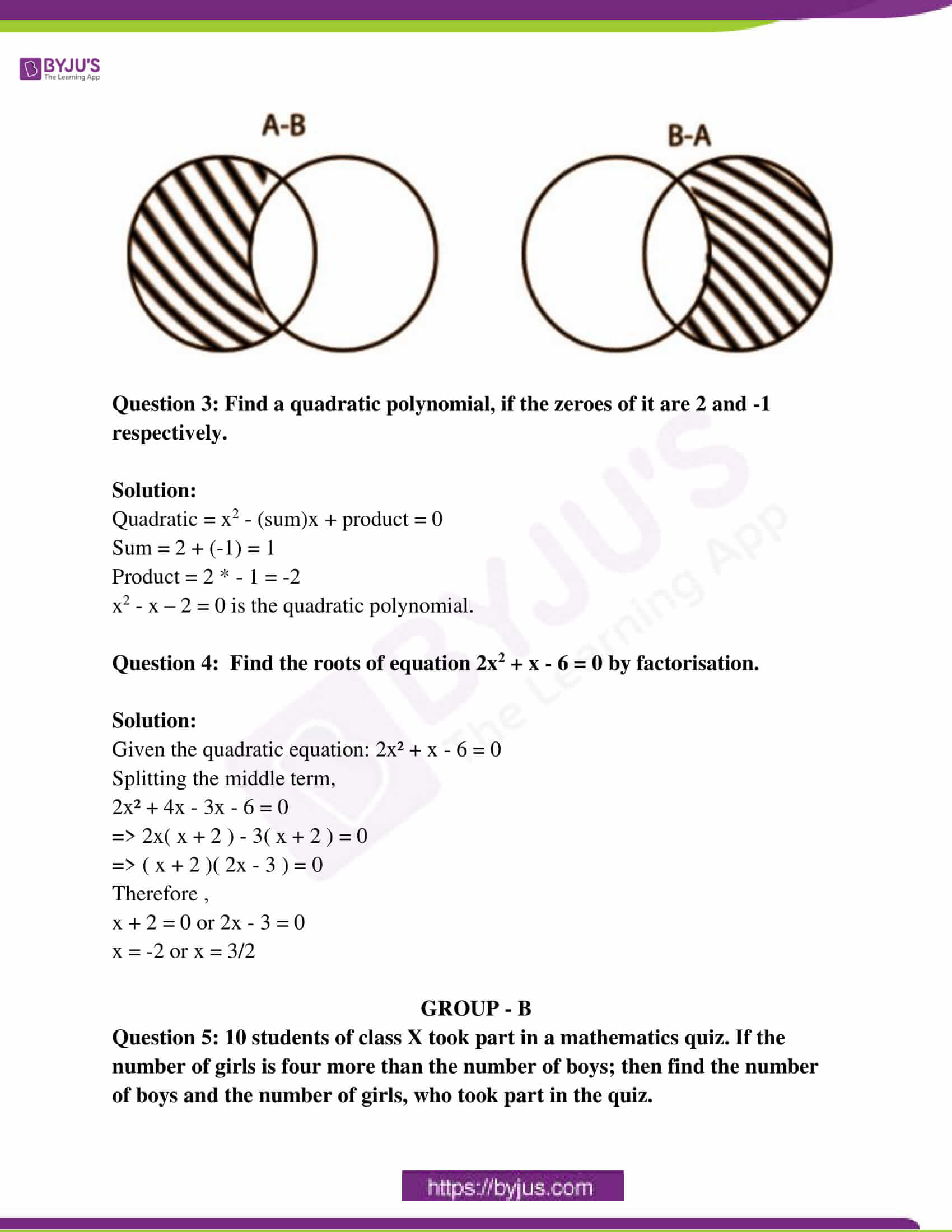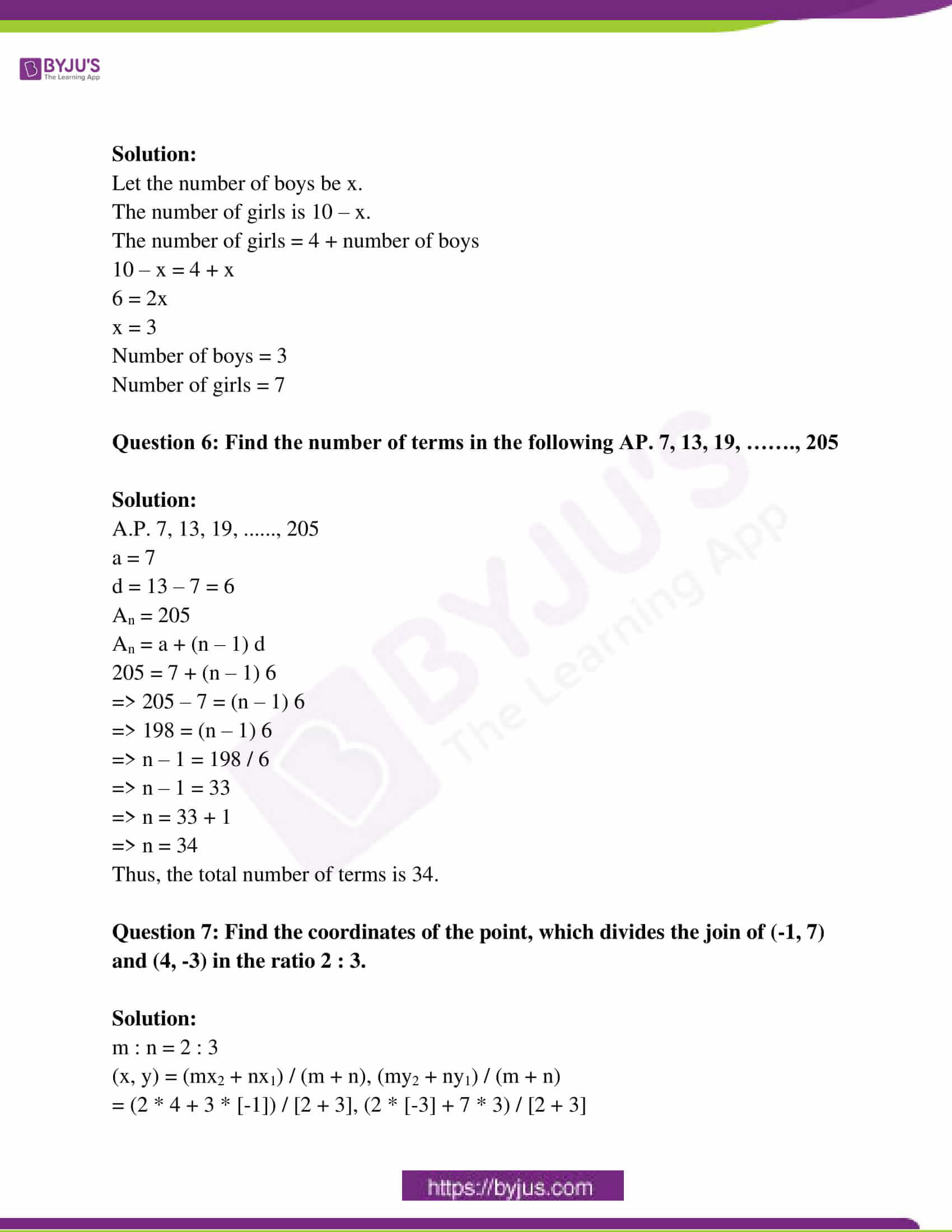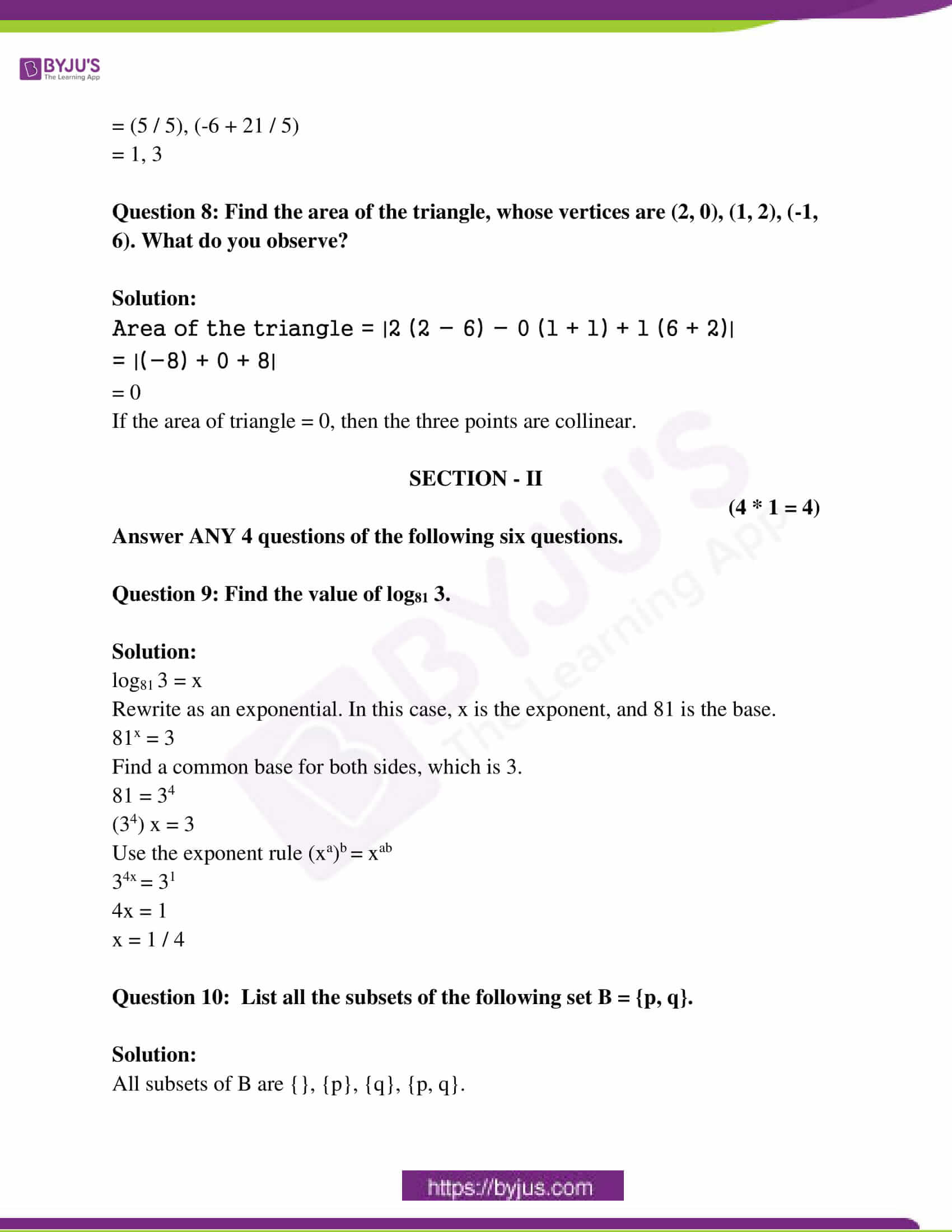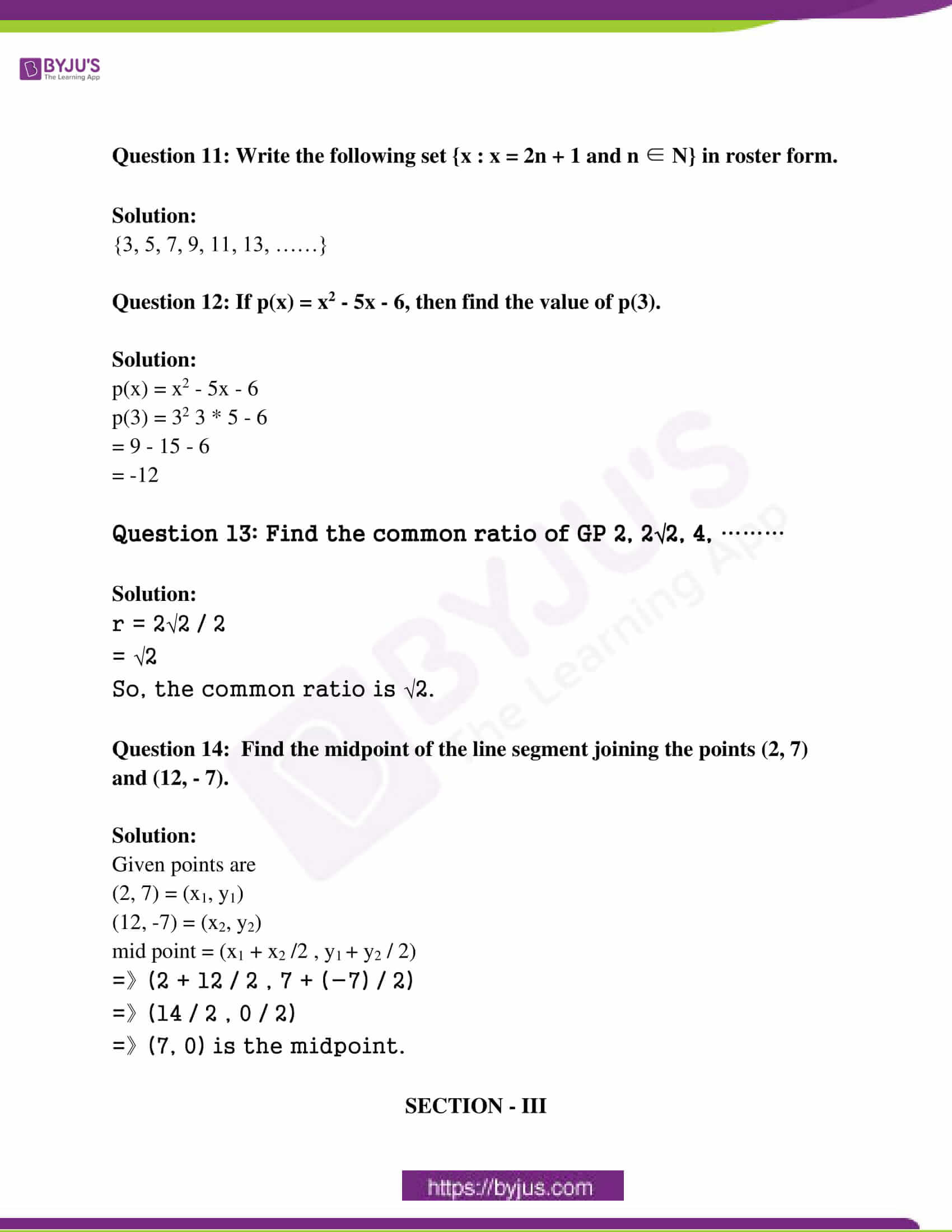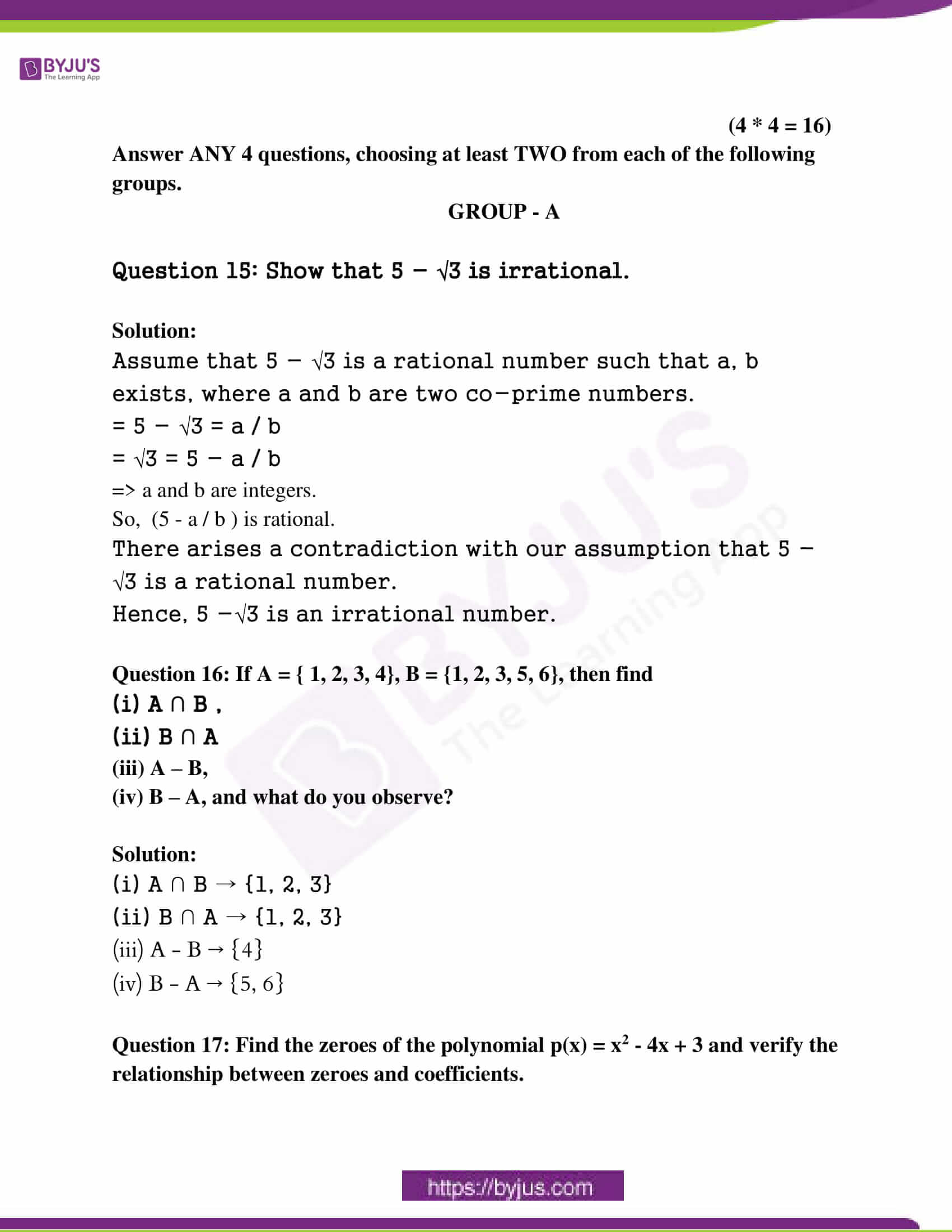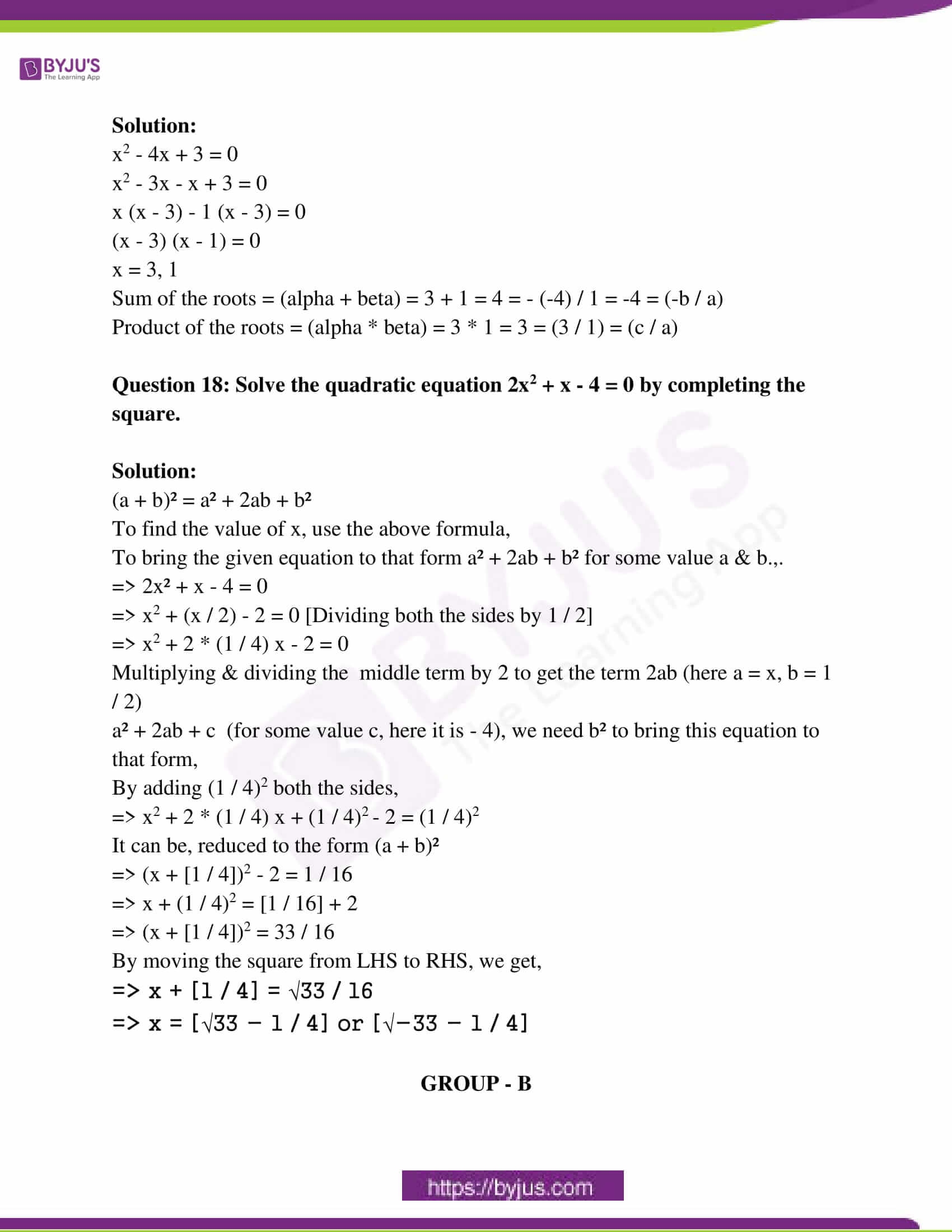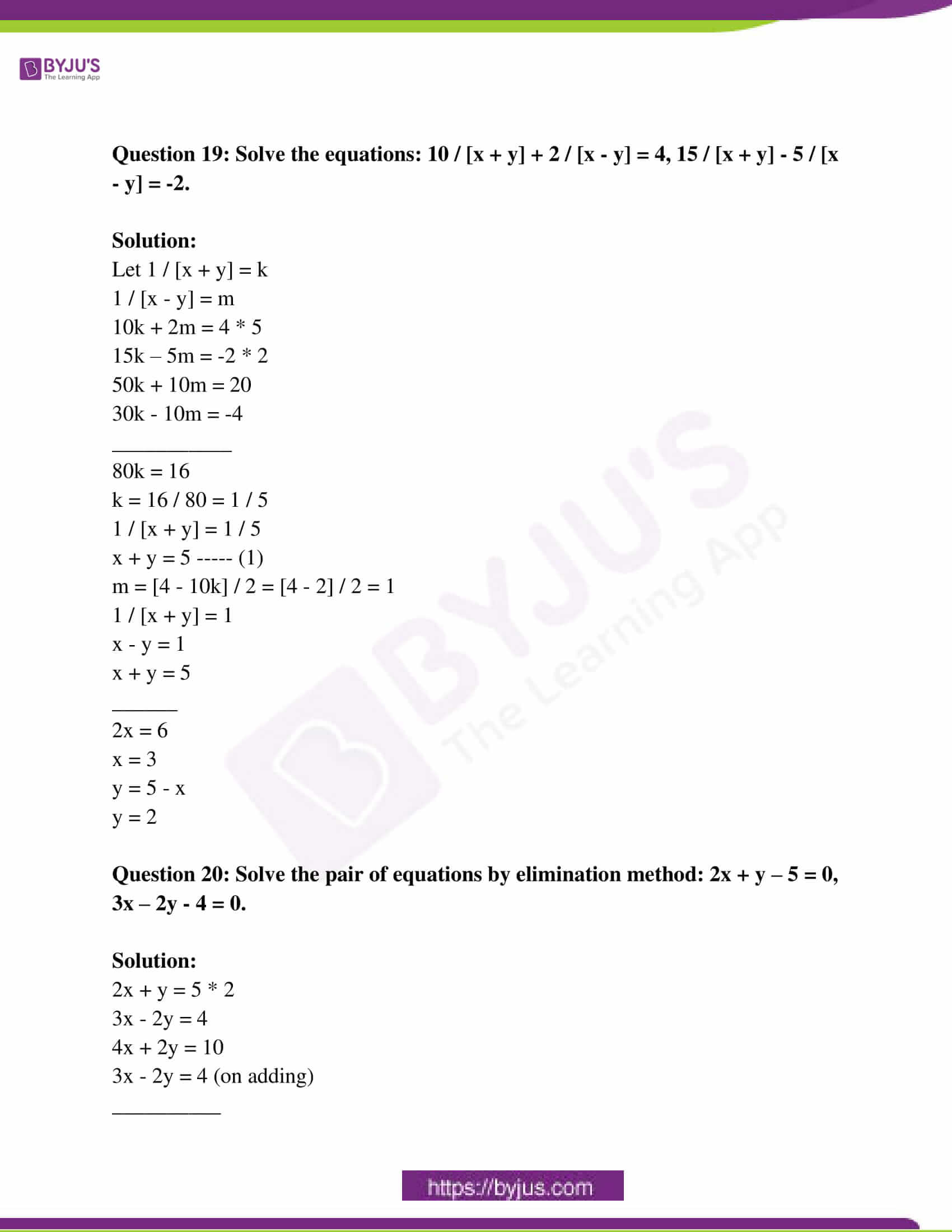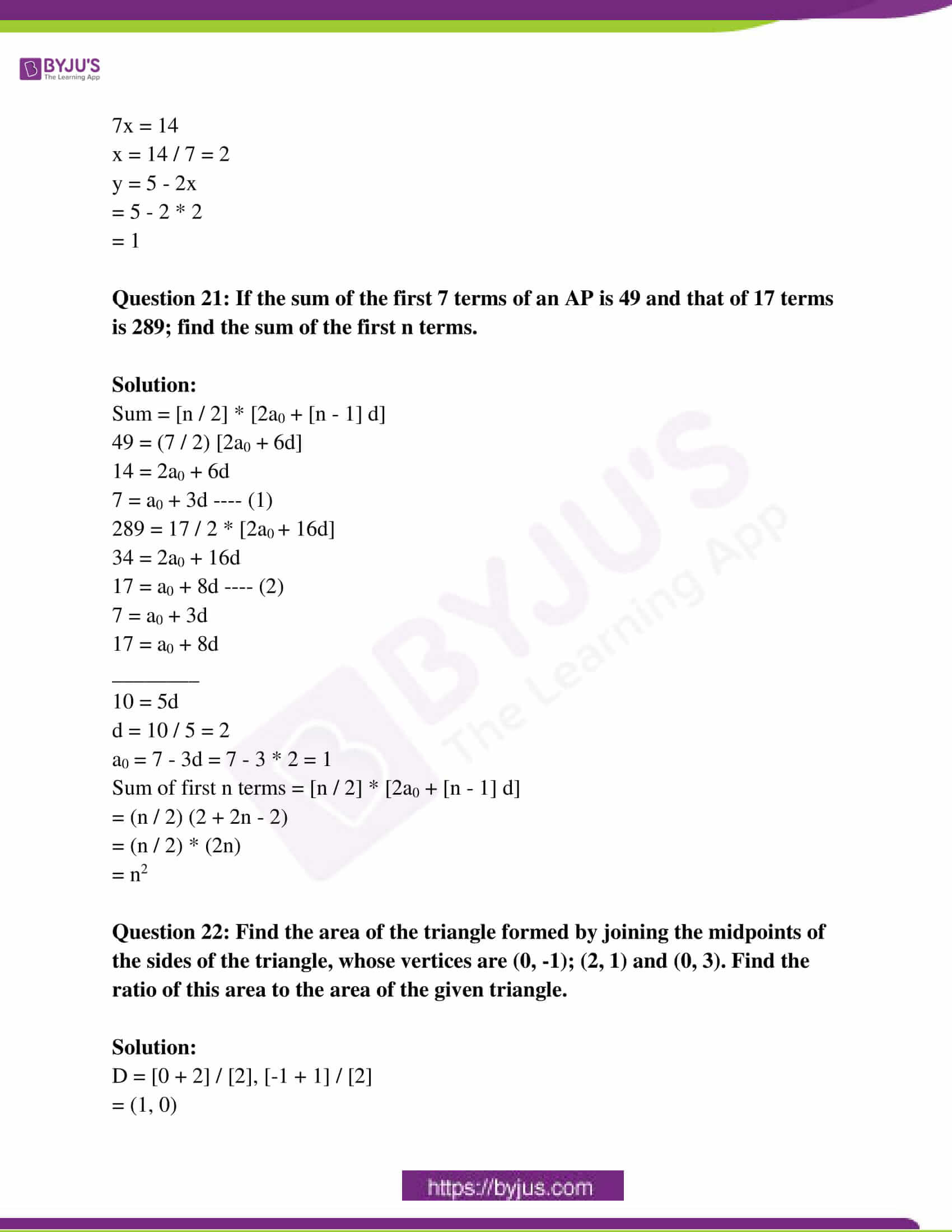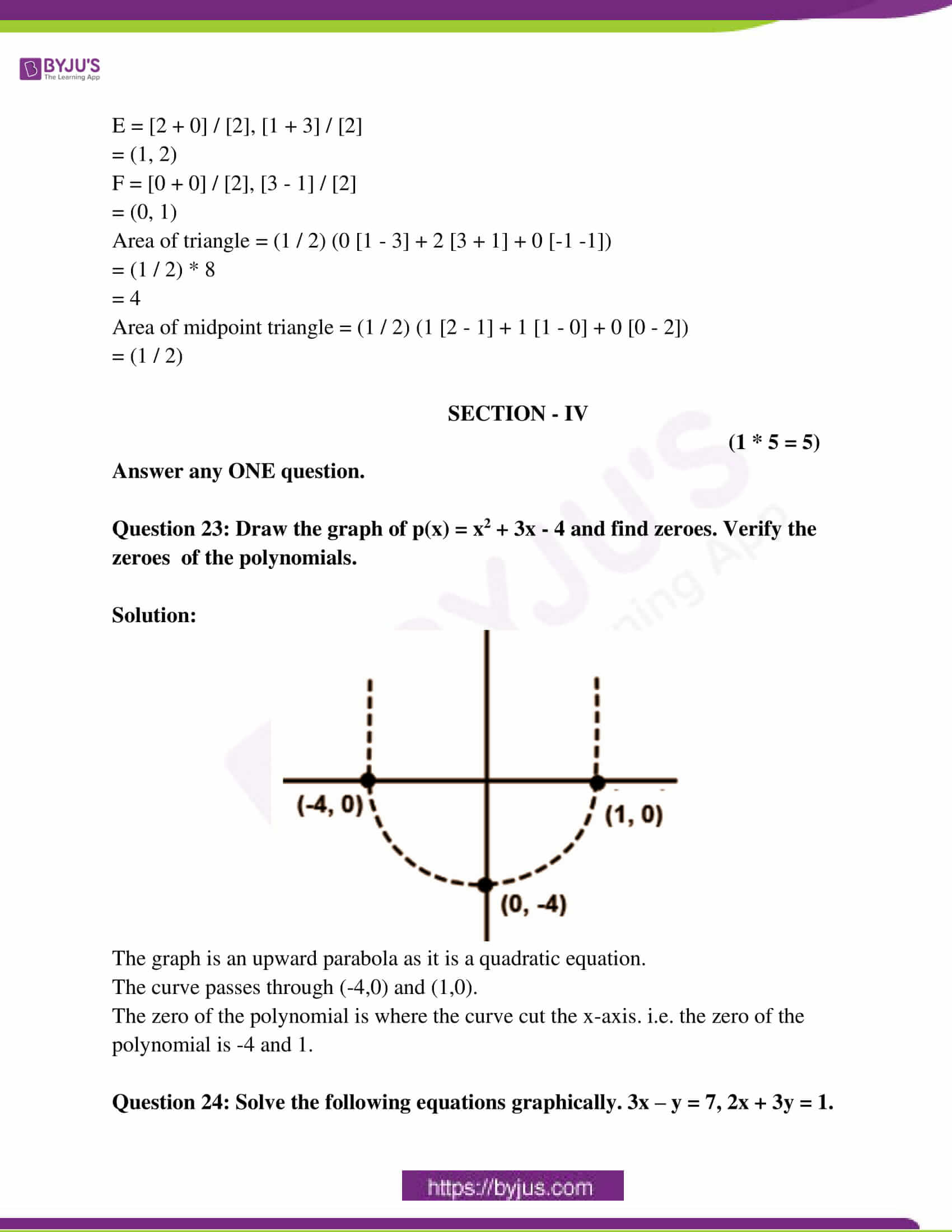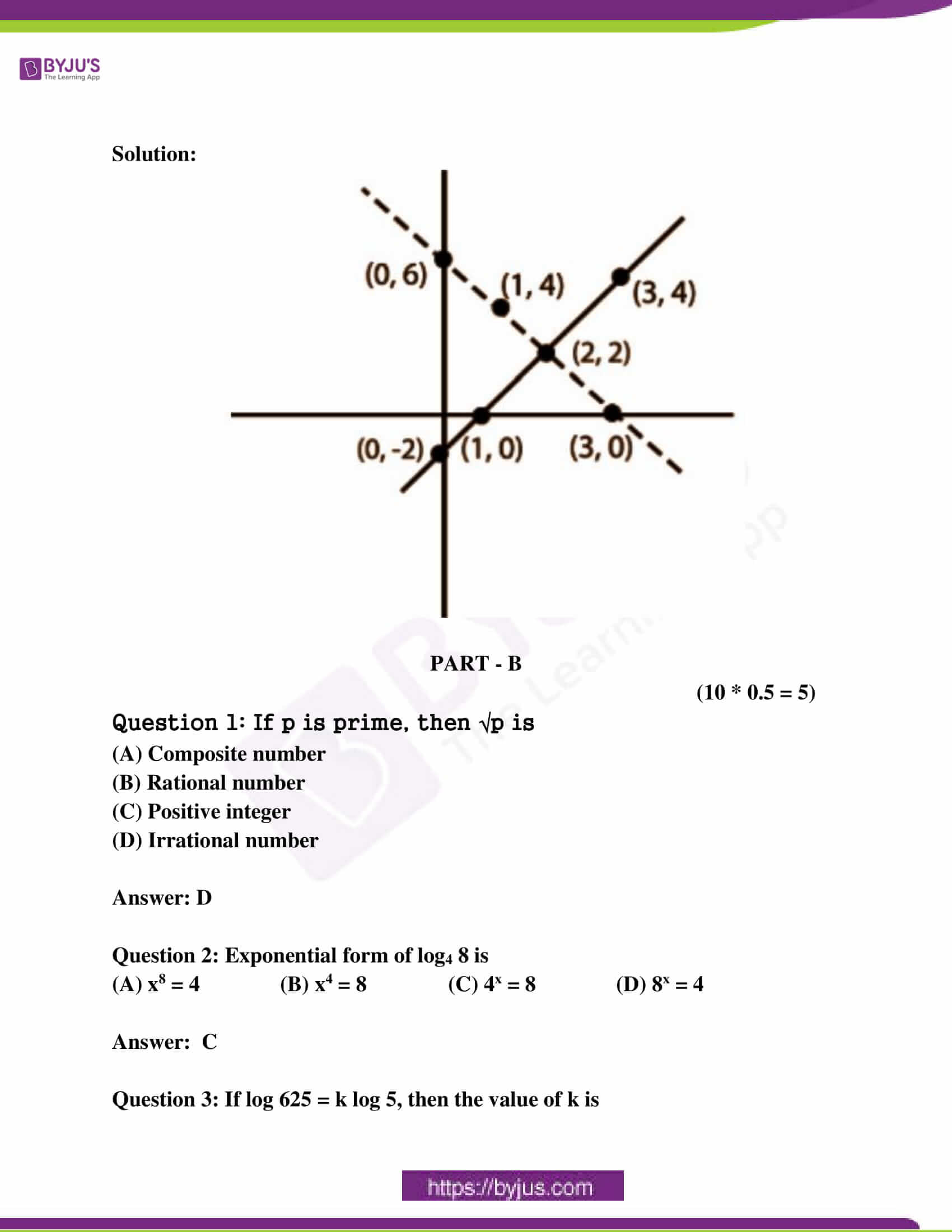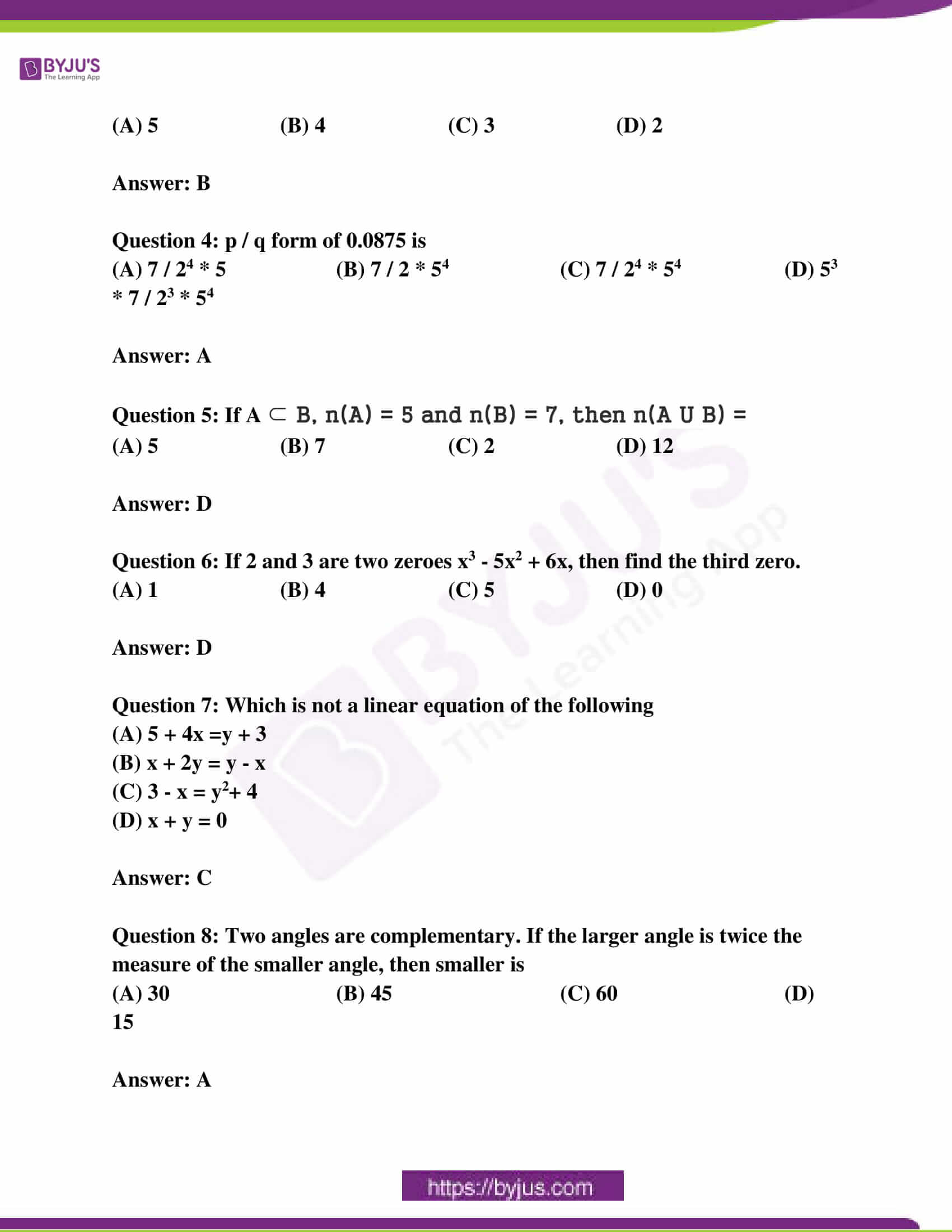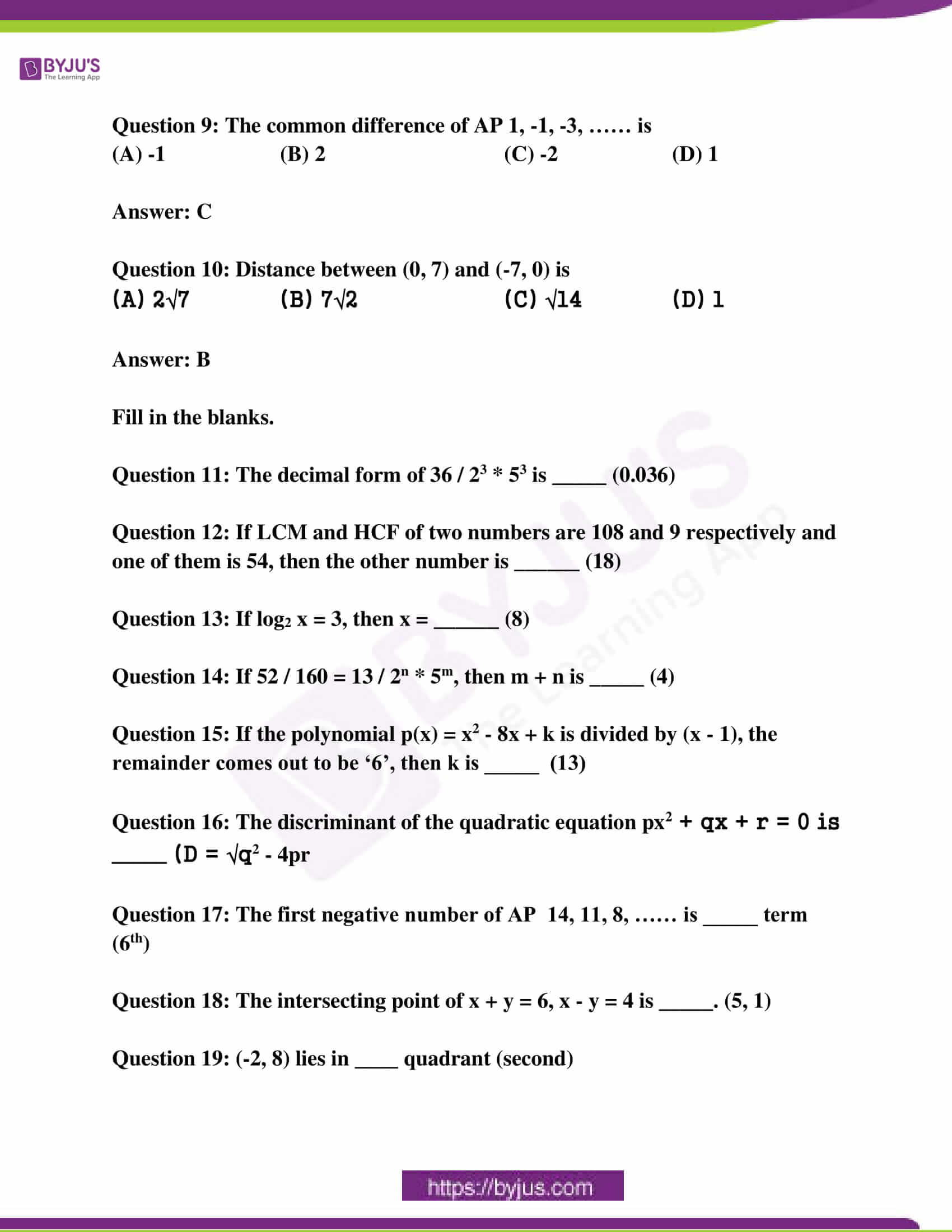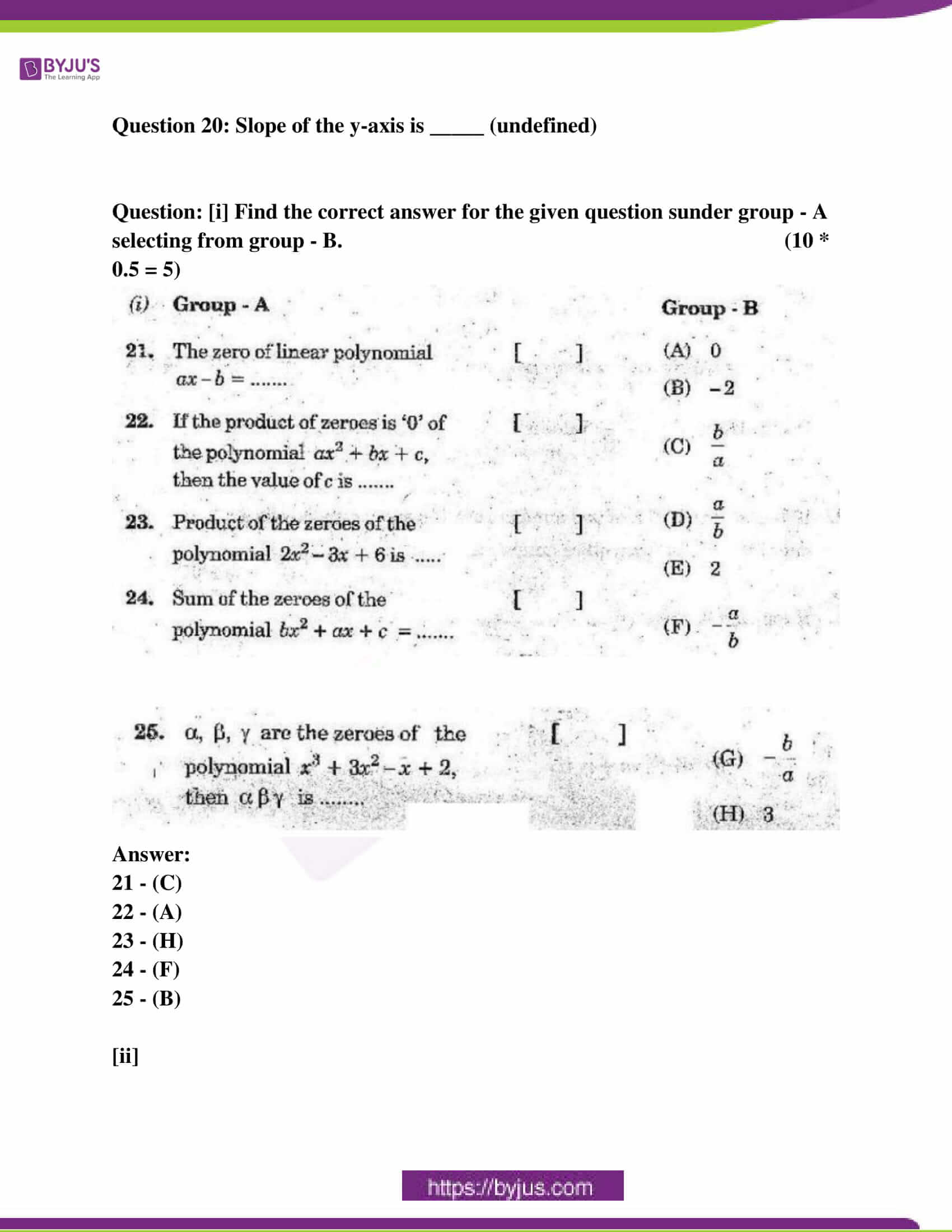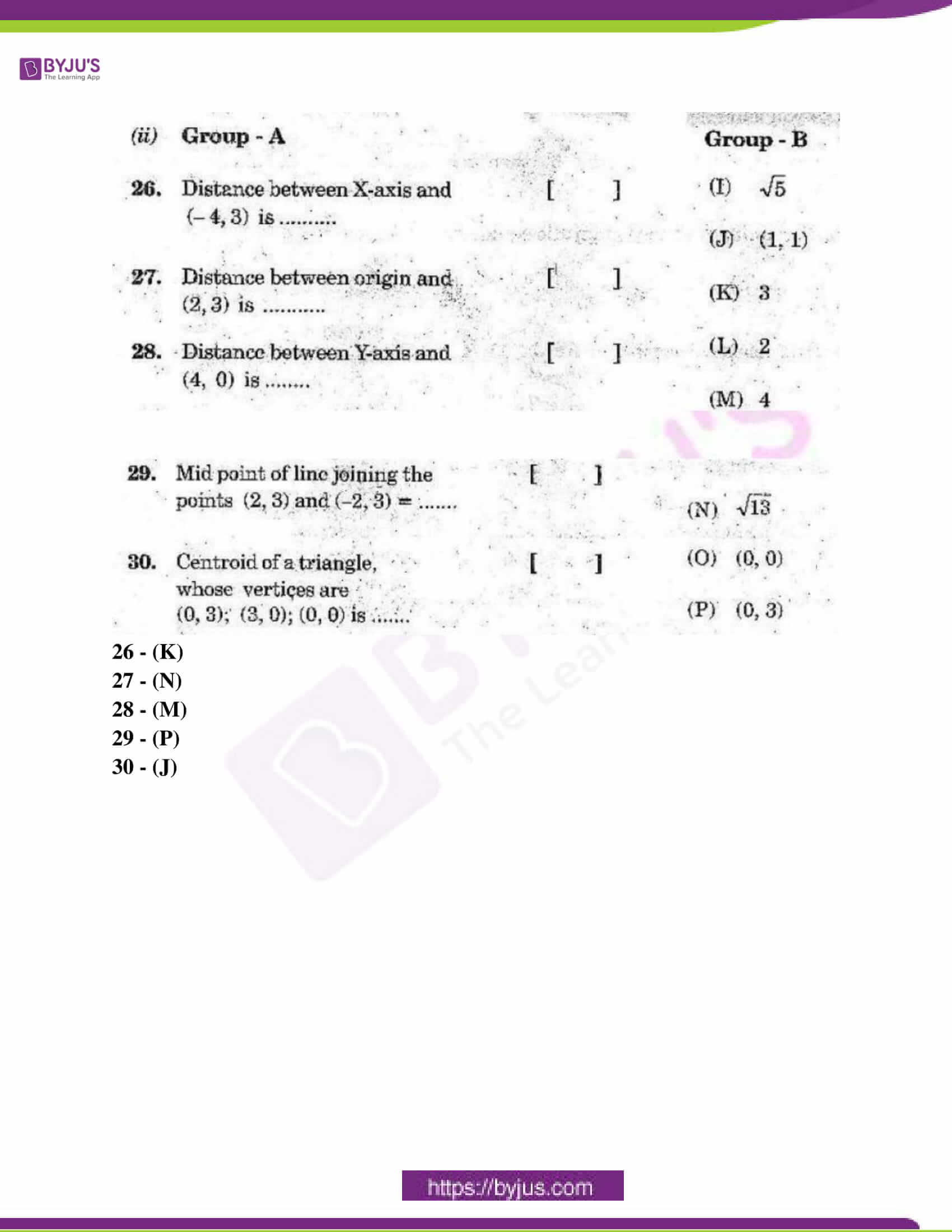SECTION – I

GROUP – A

(5 * 2 = 10)

Answer ANY 5 Questions choosing two from each of the following groups.

Question 1: Expand log 343 / 125

Solution:

Logarithmic rules:

i) log (x / y ) = log x – log y

ii) log aⁿ = n log a

log (343 / 125)

= log ( 7³/5³ )

= log ( 7 / 5 )³

= 3 log ( 7 / 5 )

= 3 [ log 7 – log 5 ]

= 3 log 7 – 3 log 5

= 3 log 7 / 3 log 5

= log 7 / log 5

Question 2: Draw the Venn diagrams of the sets (A – B), (B – A).

Solution: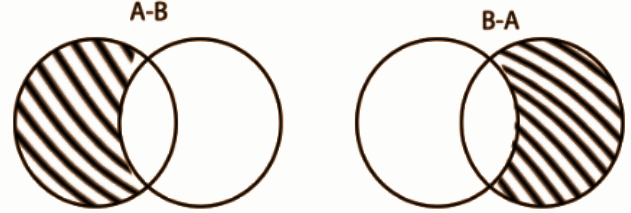Question 3: Find a quadratic polynomial, if the zeroes of it are 2 and -1 respectively.

Solution:

Quadratic = x2 – (sum)x + product = 0

Sum = 2 + (-1) = 1

Product = 2 * – 1 = -2

x2 – x – 2 = 0 is the quadratic polynomial.

Question 4: Find the roots of equation 2x2 + x – 6 = 0 by factorisation.

Solution:

Given the quadratic equation: 2x² + x – 6 = 0

Splitting the middle term,

2x² + 4x – 3x – 6 = 0

=> 2x( x + 2 ) – 3( x + 2 ) = 0

=> ( x + 2 )( 2x – 3 ) = 0

Therefore ,

x + 2 = 0 or 2x – 3 = 0

x = -2 or x = 3/2

GROUP – B

Question 5: 10 students of class X took part in a mathematics quiz. If the number of girls is four more than the number of boys; then find the number of boys and the number of girls, who took part in the quiz.

Solution:

Let the number of boys be x.

The number of girls is 10 – x.

The number of girls = 4 + number of boys

10 – x = 4 + x

6 = 2x

x = 3

Number of boys = 3

Number of girls = 7

Question 6: Find the number of terms in the following AP. 7, 13, 19, ……., 205

Solution:

A.P. 7, 13, 19, ……, 205

a = 7

d = 13 – 7 = 6

An = 205

An = a + (n – 1) d

205 = 7 + (n – 1) 6

=> 205 – 7 = (n – 1) 6

=> 198 = (n – 1) 6

=> n – 1 = 198 / 6

=> n – 1 = 33

=> n = 33 + 1

=> n = 34

Thus, the total number of terms is 34.

Question 7: Find the coordinates of the point, which divides the join of (-1, 7) and (4, -3) in the ratio 2 : 3.

Solution:

m : n = 2 : 3

(x, y) = (mx2 + nx1) / (m + n), (my2 + ny1) / (m + n)

= (2 * 4 + 3 * [-1]) / [2 + 3], (2 * [-3] + 7 * 3) / [2 + 3]

= (5 / 5), (-6 + 21 / 5)

= 1, 3

Question 8: Find the area of the triangle, whose vertices are (2, 0), (1, 2), (-1, 6). What do you observe?

Solution:

Area of the triangle = ∣2 (2 − 6) − 0 (1 + 1) + 1 (6 + 2)∣

= ∣(−8) + 0 + 8∣

= 0

If the area of triangle = 0, then the three points are collinear.

SECTION – II

(4 * 1 = 4)

Answer ANY 4 questions of the following six questions.

Question 9: Find the value of log81 3.

Solution:

log81 3 = x

Rewrite as an exponential. In this case, x is the exponent, and 81 is the base.

81x = 3

Find a common base for both sides, which is 3.

81 = 34

(34) x = 3

Use the exponent rule (xa)b = xab

34x = 31

4x = 1

x = 1 / 4

Question 10: List all the subsets of the following set B = {p, q}.

Solution:

All subsets of B are {}, {p}, {q}, {p, q}.

Question 11: Write the following set {x : x = 2n + 1 and n ∈ N} in roster form.

Solution:

{3, 5, 7, 9, 11, 13, ……}

Question 12: If p(x) = x2 – 5x – 6, then find the value of p(3).

Solution:

p(x) = x2 – 5x – 6

p(3) = 32 3 * 5 – 6

= 9 – 15 – 6

= -12

Question 13: Find the common ratio of GP 2, 2√2, 4, ………

Solution:

r = 2√2 / 2

= √2

So, the common ratio is √2.

Question 14: Find the midpoint of the line segment joining the points (2, 7) and (12, – 7).

Solution:

Given points are

(2, 7) = (x1, y1)

(12, -7) = (x2, y2)

mid point = (x1 + x2 /2 , y1 + y2 / 2)

=》(2 + 12 / 2 , 7 + (-7) / 2)

=》(14 / 2 , 0 / 2)

=》(7, 0) is the midpoint.

SECTION – III

(4 * 4 = 16)

Answer ANY 4 questions, choosing at least TWO from each of the following groups.

GROUP – A

Question 15: Show that 5 – √3 is irrational.

Solution:

Assume that 5 – √3 is a rational number such that a, b exists, where a and b are two co-prime numbers.

= 5 – √3 = a / b

= √3 = 5 – a / b

=> a and b are integers.

So, (5 – a / b ) is rational.

There arises a contradiction with our assumption that 5 – √3 is a rational number.

Hence, 5 -√3 is an irrational number.

Question 16: If A = { 1, 2, 3, 4}, B = {1, 2, 3, 5, 6}, then find

(i) A ∩ B ,

(ii) B ∩ A

(iii) A – B,

(iv) B – A, and what do you observe?

Solution:

(i) A ∩ B → {1, 2, 3}

(ii) B ∩ A → {1, 2, 3}

(iii) A – B → {4}

(iv) B – A → {5, 6}

Question 17: Find the zeroes of the polynomial p(x) = x2 – 4x + 3 and verify the relationship between zeroes and coefficients.

Solution:

x2 – 4x + 3 = 0

x2 – 3x – x + 3 = 0

x (x – 3) – 1 (x – 3) = 0

(x – 3) (x – 1) = 0

x = 3, 1

Sum of the roots = (alpha + beta) = 3 + 1 = 4 = – (-4) / 1 = -4 = (-b / a)

Product of the roots = (alpha * beta) = 3 * 1 = 3 = (3 / 1) = (c / a)

Question 18: Solve the quadratic equation 2x2 + x – 4 = 0 by completing the square.

Solution:

(a + b)² = a² + 2ab + b²

To find the value of x, use the above formula,

To bring the given equation to that form a² + 2ab + b² for some value a & b.,.

=> 2x² + x – 4 = 0

=> x2 + (x / 2) – 2 = 0 [Dividing both the sides by 1 / 2]

=> x2 + 2 * (1 / 4) x – 2 = 0

Multiplying & dividing the middle term by 2 to get the term 2ab (here a = x, b = 1 / 2)

a² + 2ab + c (for some value c, here it is – 4), we need b² to bring this equation to that form,

By adding (1 / 4)2 both the sides,

=> x2 + 2 * (1 / 4) x + (1 / 4)2 – 2 = (1 / 4)2

It can be reduced to the form (a + b)²

=> (x + [1 / 4])2 – 2 = 1 / 16

=> x + (1 / 4)2 = [1 / 16] + 2

=> (x + [1 / 4])2 = 33 / 16

By moving the square from LHS to RHS, we get,

=> x + [1 / 4] = √33 / 16

=> x = [√33 – 1 / 4] or [√-33 – 1 / 4]

GROUP – B

Question 19: Solve the equations: 10 / [x + y] + 2 / [x – y] = 4, 15 / [x + y] – 5 / [x – y] = -2.

Solution:

Let 1 / [x + y] = k

1 / [x – y] = m

10k + 2m = 4 * 5

15k – 5m = -2 * 2

50k + 10m = 20

30k – 10m = -4

___________

80k = 16

k = 16 / 80 = 1 / 5

1 / [x + y] = 1 / 5

x + y = 5 —– (1)

m = [4 – 10k] / 2 = [4 – 2] / 2 = 1

1 / [x + y] = 1

x – y = 1

x + y = 5

______

2x = 6

x = 3

y = 5 – x

y = 2

Question 20: Solve the pair of equations by elimination method: 2x + y – 5 = 0, 3x – 2y – 4 = 0.

Solution:

2x + y = 5 * 2

3x – 2y = 4

4x + 2y = 10

3x – 2y = 4 (on adding)

__________

7x = 14

x = 14 / 7 = 2

y = 5 – 2x

= 5 – 2 * 2

= 1

Question 21: If the sum of the first 7 terms of an AP is 49 and that of 17 terms is 289; find the sum of the first n terms.

Solution:

Sum = [n / 2] * [2a0 + [n – 1] d]

49 = (7 / 2) [2a0 + 6d]

14 = 2a0 + 6d

7 = a0 + 3d —- (1)

289 = 17 / 2 * [2a0 + 16d]

34 = 2a0 + 16d

17 = a0 + 8d —- (2)

7 = a0 + 3d

17 = a0 + 8d

________

10 = 5d

d = 10 / 5 = 2

a0 = 7 – 3d = 7 – 3 * 2 = 1

Sum of first n terms = [n / 2] * [2a0 + [n – 1] d]

= (n / 2) (2 + 2n – 2)

= (n / 2) * (2n)

= n2

Question 22: Find the area of the triangle formed by joining the midpoints of the sides of the triangle, whose vertices are (0, -1); (2, 1) and (0, 3). Find the ratio of this area to the area of the given triangle.

Solution:

D = [0 + 2] / , [-1 + 1] / 

= (1, 0)

E = [2 + 0] / , [1 + 3] / 

= (1, 2)

F = [0 + 0] / , [3 – 1] / 

= (0, 1)

Area of triangle = (1 / 2) (0 [1 – 3] + 2 [3 + 1] + 0 [-1 -1])

= (1 / 2) * 8

= 4

Area of midpoint triangle = (1 / 2) (1 [2 – 1] + 1 [1 – 0] + 0 [0 – 2])

= (1 / 2)

SECTION – IV

(1 * 5 = 5)

Question 23: Draw the graph of p(x) = x2 + 3x – 4 and find zeroes. Verify the zeroes of the polynomials.

Solution: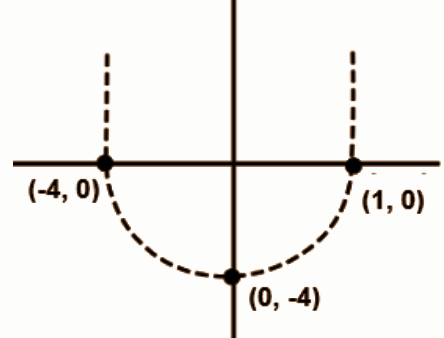The graph is an upward parabola as it is a quadratic equation.

The curve passes through (-4,0) and (1,0).

The zero of the polynomial is where the curve cut the x-axis. i.e. the zero of the polynomial is -4 and 1.

Question 24: Solve the following equations graphically. 3x – y = 7, 2x + 3y = 1.

Solution: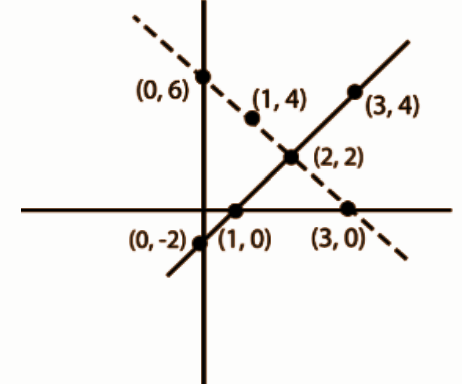PART – B

(10 * 0.5 = 5)

Question 1: If p is prime, then √p is

(A) Composite number

(B) Rational number

(C) Positive integer

(D) Irrational number

Question 2: Exponential form of log4 8 is

(A) x8 = 4 (B) x4 = 8 (C) 4x = 8 (D) 8x = 4

Question 3: If log 625 = k log 5, then the value of k is

(A) 5 (B) 4 (C) 3 (D) 2

Question 4: p / q form of 0.0875 is

(A) 7 / 24 * 5 (B) 7 / 2 * 54 (C) 7 / 24 * 54 (D) 53 * 7 / 23 * 54

Question 5: If A ⊂ B, n(A) = 5 and n(B) = 7, then n(A U B) =

(A) 5 (B) 7 (C) 2 (D) 12

Question 6: If 2 and 3 are two zeroes x3 – 5x2 + 6x, then find the third zero.

(A) 1 (B) 4 (C) 5 (D) 0

Question 7: Which is not a linear equation of the following

(A) 5 + 4x =y + 3

(B) x + 2y = y – x

(C) 3 – x = y2+ 4

(D) x + y = 0

Question 8: Two angles are complementary. If the larger angle is twice the measure of the smaller angle, then smaller is

(A) 30 (B) 45 (C) 60 (D) 15

Question 9: The common difference of AP 1, -1, -3, …… is

(A) -1 (B) 2 (C) -2 (D) 1

Question 10: Distance between (0, 7) and (-7, 0) is

(A) 2√7 (B) 7√2 (C) √14 (D) 1

Fill in the blanks.

Question 11: The decimal form of 36 / 23 * 53 is _____ (0.036)

Question 12: If LCM and HCF of two numbers are 108 and 9, respectively and one of them is 54, then the other number is ______ (18)

Question 13: If log2 x = 3, then x = ______ (8)

Question 14: If 52 / 160 = 13 / 2n * 5m, then m + n is _____ (4)

Question 15: If the polynomial p(x) = x2 – 8x + k is divided by (x – 1), the remainder comes out to be ‘6’, then k is _____ (13)

Question 16: The discriminant of the quadratic equation px2 + qx + r = 0 is _____ (D = √q2 – 4pr

Question 17: The first negative number of AP 14, 11, 8, …… is _____ term (6th)

Question 18: The intersecting point of x + y = 6, x – y = 4 is _____. (5, 1)

Question 19: (-2, 8) lies in ____ quadrant (second)

Question 20: Slope of the y-axis is _____ (undefined)

Question: [i] Find the correct answer for the given questions under group – A selecting from group – B. (10 * 0.5 = 5)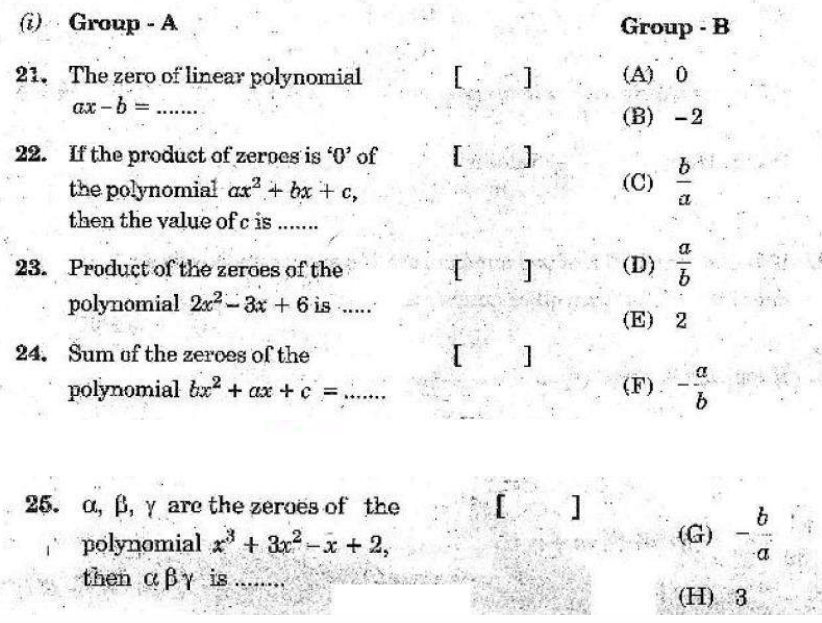21 – (C)

22 – (A)

23 – (H)

24 – (F)

25 – (B)

[ii]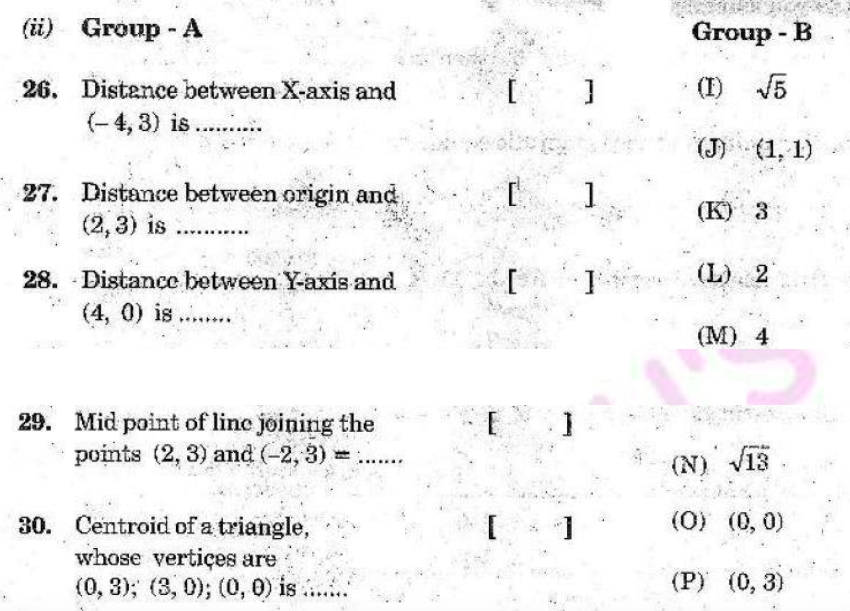26 – (K)

27 – (N)

28 – (M)

29 – (P)

30 – (J)